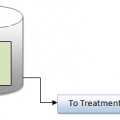## Why Hypochlorite Consumption is higher than Chlorine?

In connection with previous post. let we see, why? hypochlorite consumption is always higher than that chlorine gas consumption.this is very simple calculation involves.

Generally the hypochlorite is hazardous than chlorine it is similar to strong bleach and available in two forms one is Dry HTH second as Liquid Sodium Hypochlorite.

In HTH forms the available chlorine normally about 60-65% but sodium hypochlorite contains about 11 to 15% available chlorine (in industrial strengths).

Key note : Because either type of hypochlorite is not 100% pure chlorine, due to this reason we want use higher dosage fed into same disinfection or color removal Some studies indicate that such a substitution can increase operating costs, overall, by up to 3- 5 times the cost of using chlorine.

Now let we see with simple example:

A total chlorine dosage of 10 mg/L is required to treat a particular wastewater. If the flow is 1.4 MGD and the hypochlorite has 65% available chlorine how many kg/d of hypochlorite will be required? (MGD=Million Gallon Day)(3.78kg/Gallon)

Solution:
Step 1: Calculate the kg/day chlorine required using the mg/L to kg/d equation:

mg/L x MGD x 3.78 = kg/d

10 mg/L x 1.4 MGD x 3.78 kg/g= 52.92 kg/g (say 53 kg/day)

Step 2: Calculate the kg/d hypochlorite required. Since only 65% of the hypochlorite is chlorine, more than 117 lb/d will be required:

53 kg/day Cl / 65% Available Cl x 100 = 81.53 kg/d hypochlorite is require

Now,You might very clear..## Author:T.P.Sivanandan

#### Why Fine Bubbles are Better than Coarse bubble in Aeration Tank#### How to Calculate Lime Dosage Requirement in Sedimentation Process